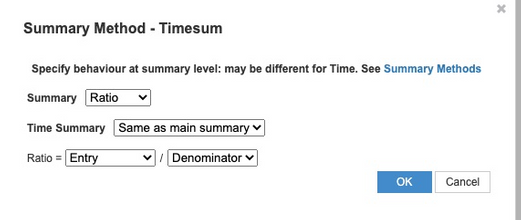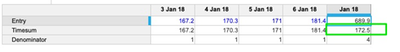# TIMESUM Function with aggregation method AVERAGEHi,

I am using TIMESUM function with AVERAGE as aggregation method for specific from-to period. Some of the cells have the zero values in it, but this functions is considering those zero cells also into count . This gives me wrong average. How can we skip zero cells and calculate average only for non zero values with specific time period range. See attached example average of below with TIMESUM function is showing as 115 instead of 172.5.  I hope someone can help me here. Thanks in advance.

Best Regards,

Suman.

Tagged:

•Can you do this using the Ratio Summary Method?

I have 3 line items:

Entry: This is equal to the line in your screenshot

Timesum: This is the target line item where you are doing your timesum, and need your summary to be 172.5

Denominator: This is just counting the number of entries that we have that are not 0. So in this case the Formula says: IF Entry <> 0 THEN 1 ELSE 0

In the summary of the Timesum line, I have this:I hope this helps!
Jason

•Hi Jason,

Thank you for the suggestion. But  i have to get the average only with TIMESUM() function instead of using summary methods. Because i do not have time dimension in result module.

Best Regards,

Suman.

•Hi Suman,

Ratio is independent of time. You can use it in a module which doesn't have a time dimension as well.

@jasonblinn Has given an example for reference.

Alternative method if you want to go for timesum specifically.

Create one lineitem say Check ,boolean formatted; formula : If X>0 then true else false.[X is the line item with values]

Now, for Timesum lineitem :- If check then Timesum(,,,,) else 0

Hope this helps

~Abhi